# 程序员最近都爱上了这个网站  程序员们快来瞅瞅吧！  it98k网:it98k.com

出租广告位,需要合作请联系站长

+关注

2022-10(40)

2022-11(70)

2022-12(9)

2023-01(26)

2023-02(10)

## 告别手敲 SQL ？GPT-3 自动帮你写#【导语】手写业务 SQL 很繁琐？GPT-3来帮你！本文作者通过手动输入简单的英文描述秒 Get 到 SQL 了。听说 AI 又来抢开发者饭碗，一起来看看吧：

GPT-3 是一种文本完成引擎，它接受输入的文本并返回它认为接下来会出现的文本。现在已经有很多人使用它根据指定说明来生成 HTML、CSS 等代码。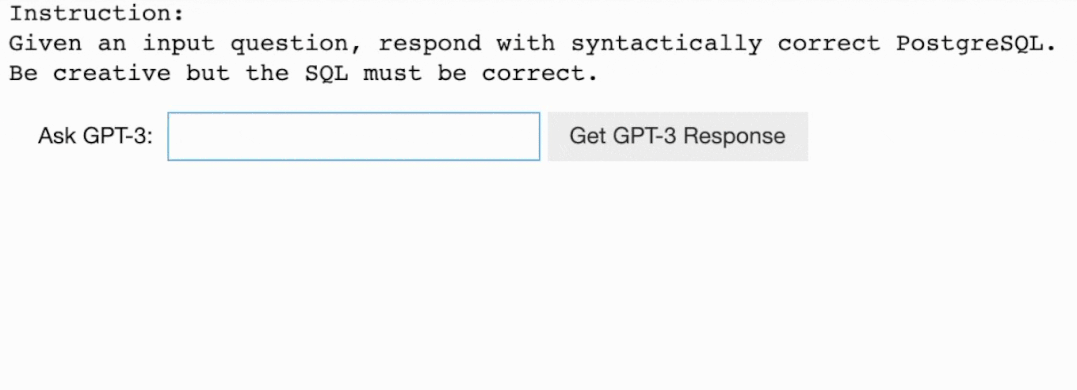GPT-3：

``SELECT COUNT(*) FROM usersWHERE signup_time > now() - interval '1 month'``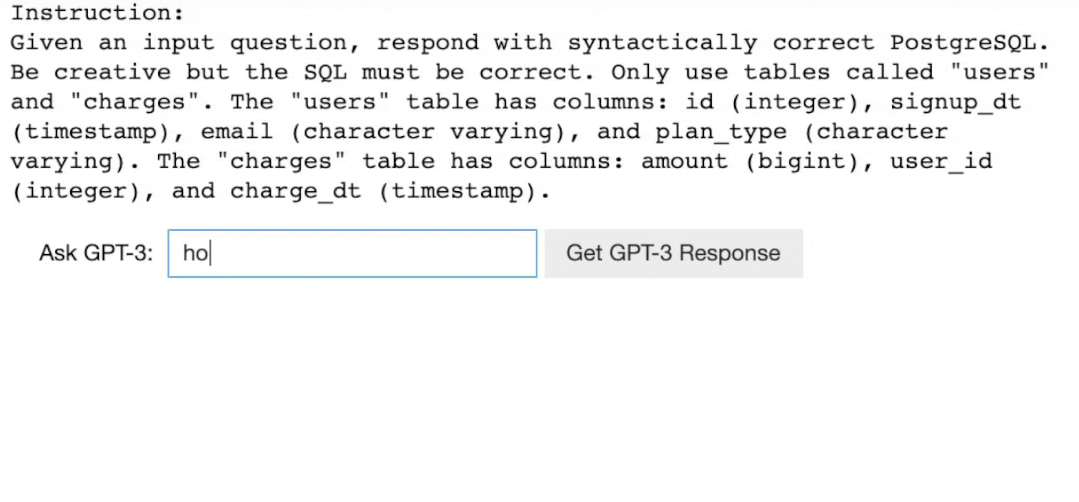GPT-3：

``````SELECT SUM(amount) FROM charges WHERE charge_dt > now() - interval '7 days'
``````### 用示例启动GPT-3

``````SELECT id, signup_dt, email, plan_type FROM users
``````

``````SELECT amount, user_id, and charge_dt FROM charges
``````

将这些示例提供给 GPT-3，我将再问一个问题：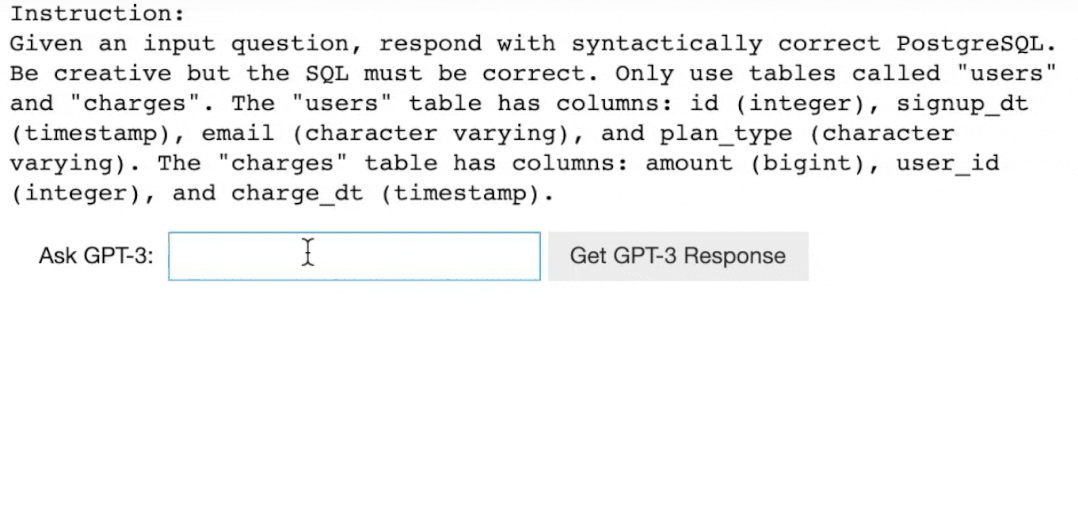GPT-3：

``````SELECT MAX(amount) FROM charges WHERE charge_dt > NOW() - INTERVAL '30 days'
``````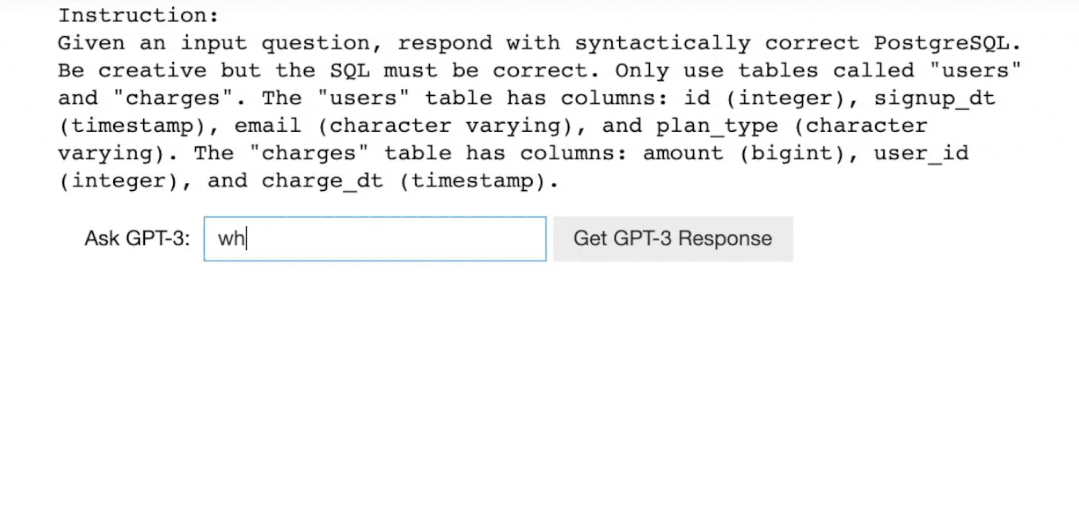GPT-3：

``````SELECT MAX(charge_dt) FROM charges WHERE user_id = 1 AND email = 'brian@seekwell.io'
``````

``SELECT MIN(charge_dt) as last_payment_dt from charges LEFT JOINusers ON users.id = charges.user_idWHERE users.email = 'jane@seekwell.io'``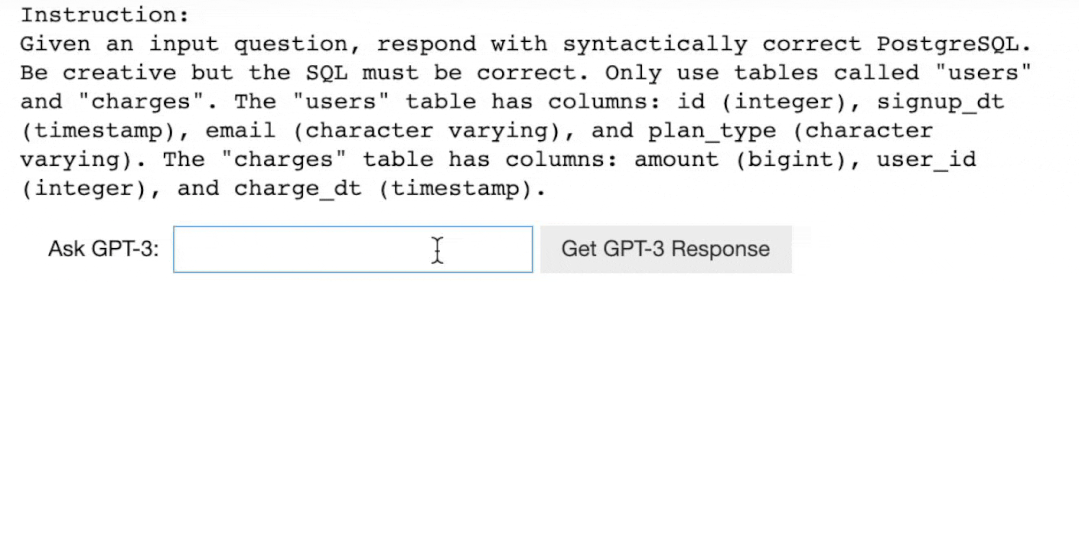GPT-3：

``````SELECT MAX(charge_dt) as last_payment_dt from charges LEFT JOIN users ON users.id = charges.user_id WHERE users.email = 'brian@seekwell.io'
``````

``````SELECT COUNT(*) FROM users WHERE signup_dt >= now() - interval '30 days'
``````

``````SELECT signup_dt FROM users WHERE email = 'brian@seekwell.io'
``````

``````SELECT SUM(amount) from charges WHERE charge_dt >= now() - interval '7 days'
``````

``SELECT SUM(case when charge_dt>= '10-01-20'::date and charge_dt < '11-15-20'::date then amount else 0 end) as revenueFROM charges``

``SELECT SUM(charges.amount) FROM users INNER JOINcharges ON users.id = charges.user_idWHERE users.signup_dt>= now() - interval '6 months'``

``SELECT MIN(charge_dt) as last_payment_dt from users INNER JOINcharges ON users.id = charges.user_idWHERE users.email = 'brian@seekwell.io'``

``SELECT sum(case when signup_dt>= now() - interval '1 month' then 1 else 0 end) as signups_this_month,sum(case when signup_dt>= now() - interval '2 months' and signup_dt < now() - interval '1 month'  then 1 else 0 end) as signups_last_monthFROM users``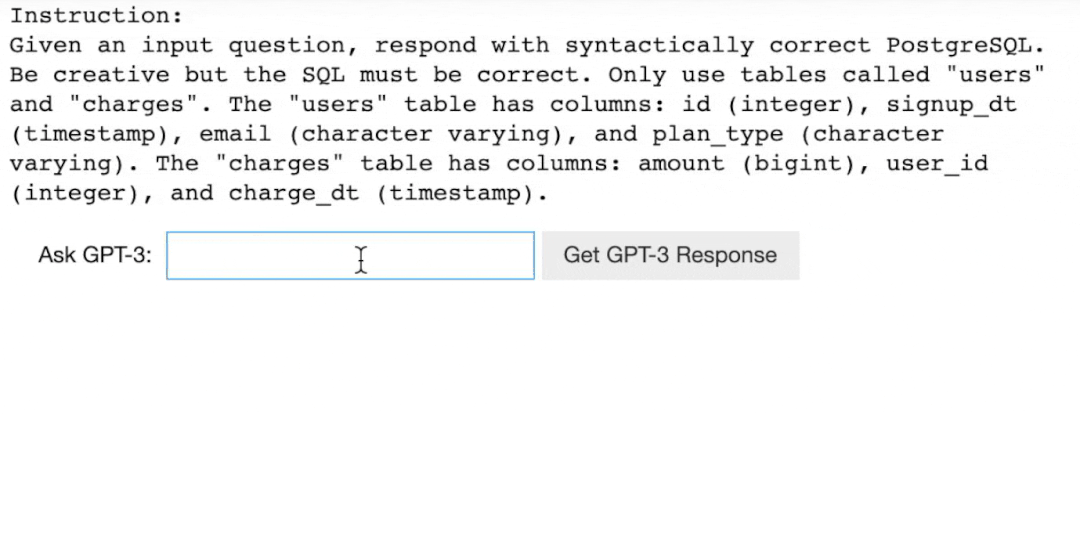GPT-3：

``````SELECT COUNT(*) FROM users INNER JOIN  charges ON users.id = charges.user_id WHERE users.email = 'joe@freshpizza.com'
``````

GPT-3认为这里的COUNT（）是适当的表达式，知道将“用户”与“收费”表结合在一起，并将电子邮件正确设置为我所询问的电子邮件。GPT-3的另一个问题：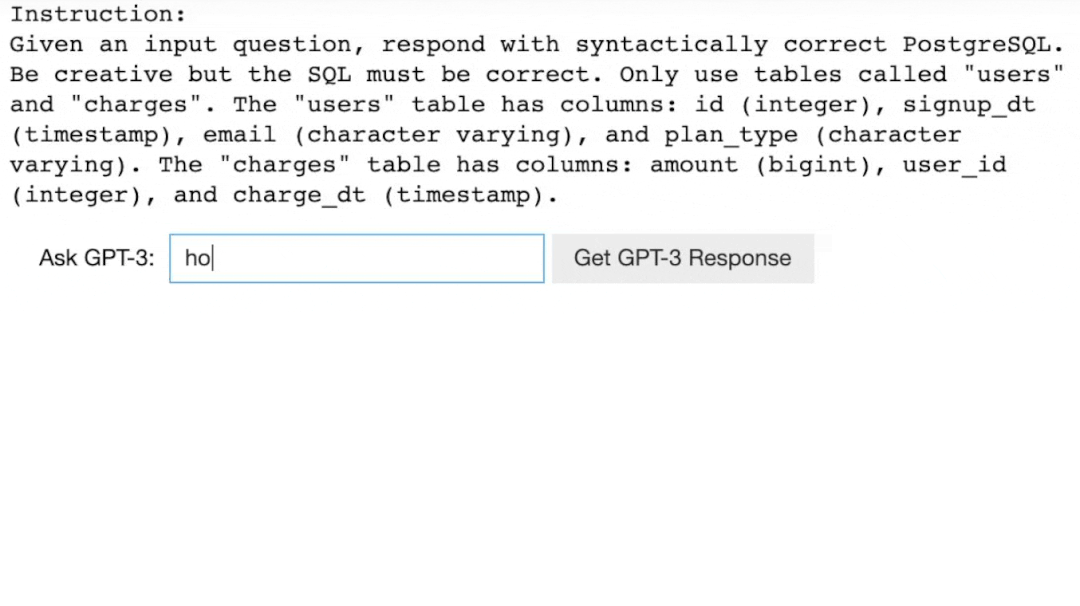GPT-3：

``````SELECT SUM(charges.amount) FROM users INNER JOIN charges ON users.id = charges.user_id WHERE users.email LIKE '%seekwell.io%' AND users.signup_dt>= now() - interval '3 months'
``````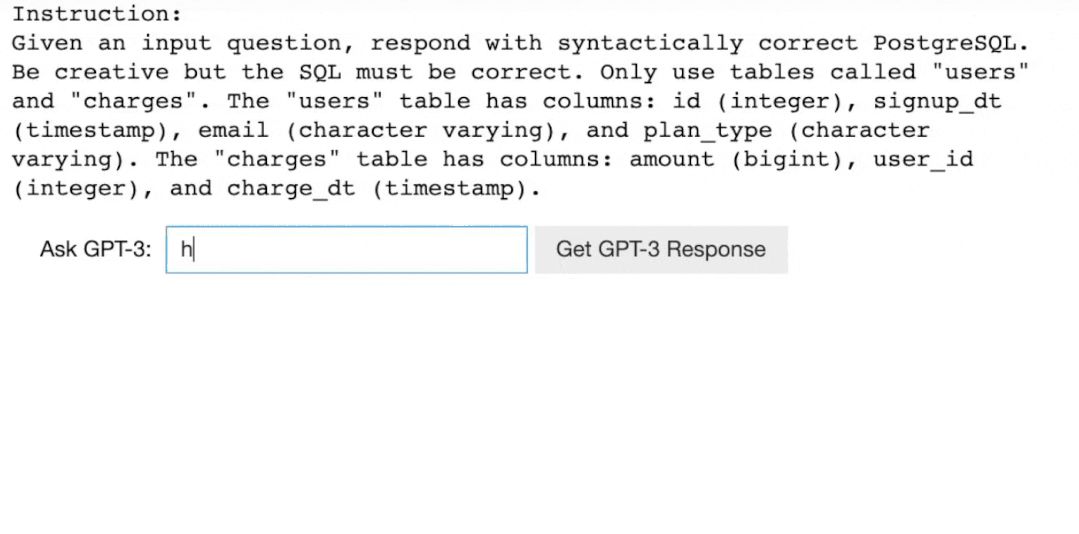GPT-3：

``````SELECT SUM(case when charge_dt >= '06-01-2020'::date and charge_dt < '08-01-2020'::date then amount else 0 end) as revenue FROM charges
``````### 将 GPT-3 “玩坏了”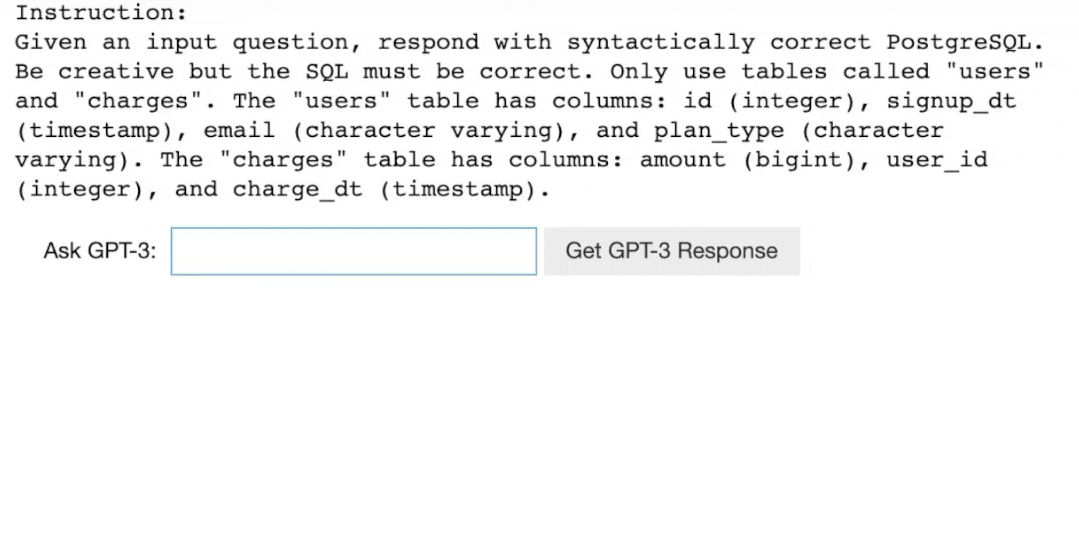GPT-3：

``````SELECT COUNT() as total_users, COUNT() as total_paid_users, 100.0*(COUNT() as total_paid_users) / COUNT() as total_users AS percent_converted FROM users
``````

``SELECT SUM(CASE WHEN email like '%gmail.com' then 1 else 0 end)/COUNT(*) as percent_gmailFROM users``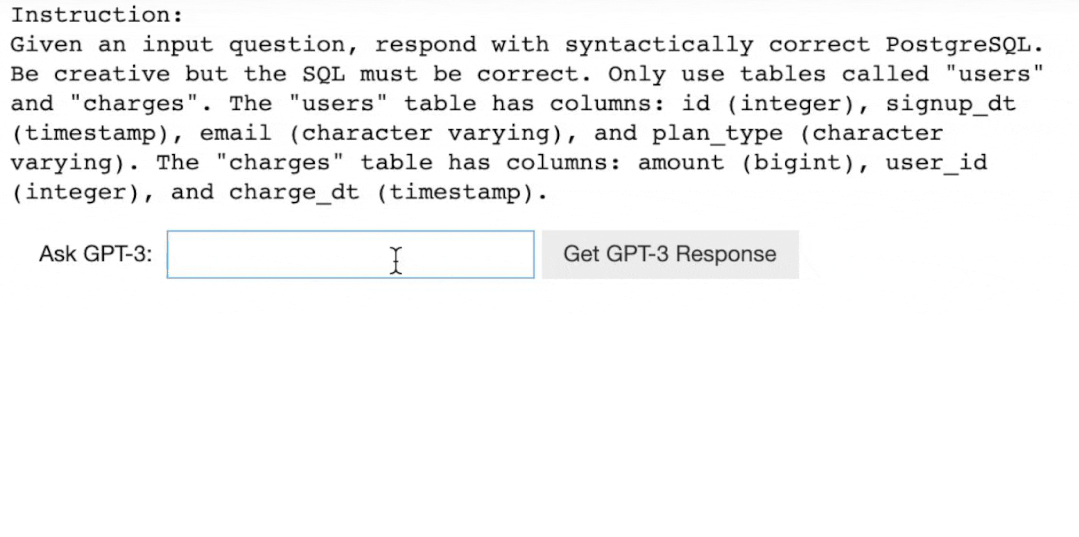GPT-3：

``````SELECT SUM(CASE WHEN plan_type = 'pro' THEN 1 else 0 end)/COUNT(*) as percent_paid FROM users
``````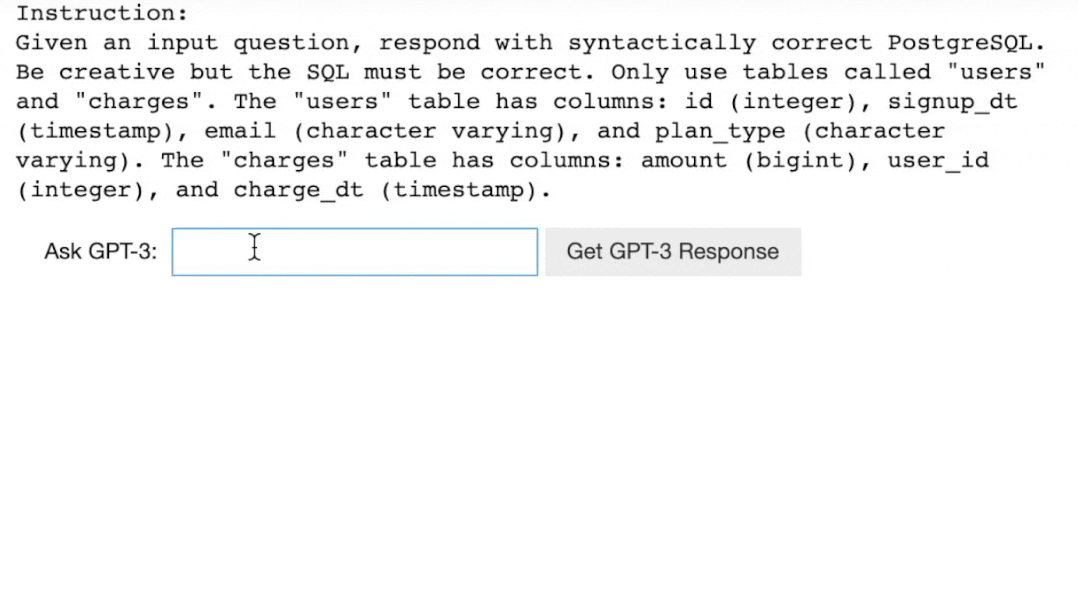GPT-3：

``````SELECT SUM(CASE WHEN plan_type='pro' THEN 1 ELSE 0 END)/COUNT(*) AS percent_paid FROM users WHERE users.signup_dt>= now() - interval '6 months'
``````### 调整 GPT-3 的参数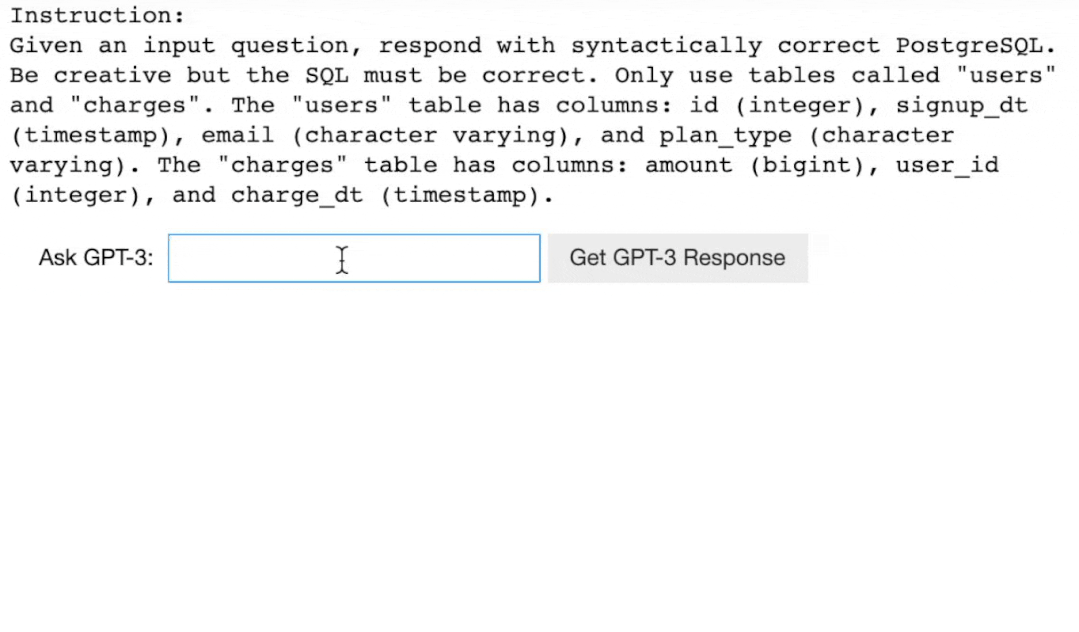Temperature为.8时 GPT-3：

``````SELECT SUM(case when charge_dt>= now() - interval '1 month'  then charges.amount else 0 end) as revenue_this_month, CASE WHEN signup_dt>= now() - interval '2 months' AND signup_dt < now() - interval '1 month' THEN SUM(charges.amount) else 0 end as revenue_last_month FROM charges  LEFT OUTER JOIN users ON users.id = charges.user_id \ WHERE users.signup_dt >= now() - interval '1 month'
``````Temperature为.3时 GPT-3：

``SELECT SUM(case when charge_dt>= now() - interval '1 month' then amount else 0 end) as revenue_this_month, SUM(case when charge_dt>= now() - interval '2 months' and charge_dt < now() - interval '1 month' then amount else 0 end) as revenue_last_month FROM charges ``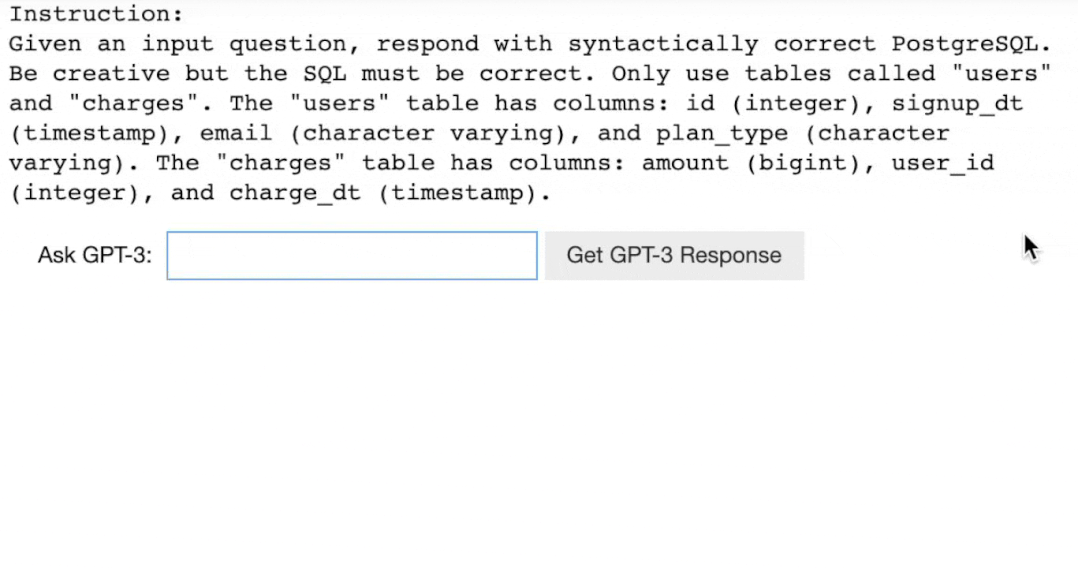Temperature为.8时 GPT-3：

``SELECT SUM(charges.amount) FROM users INNER JOIN charges ON users.id = charges.user_id WHERE signup_dt >= DATE_SUB(now(), INTERVAL '6 months') ``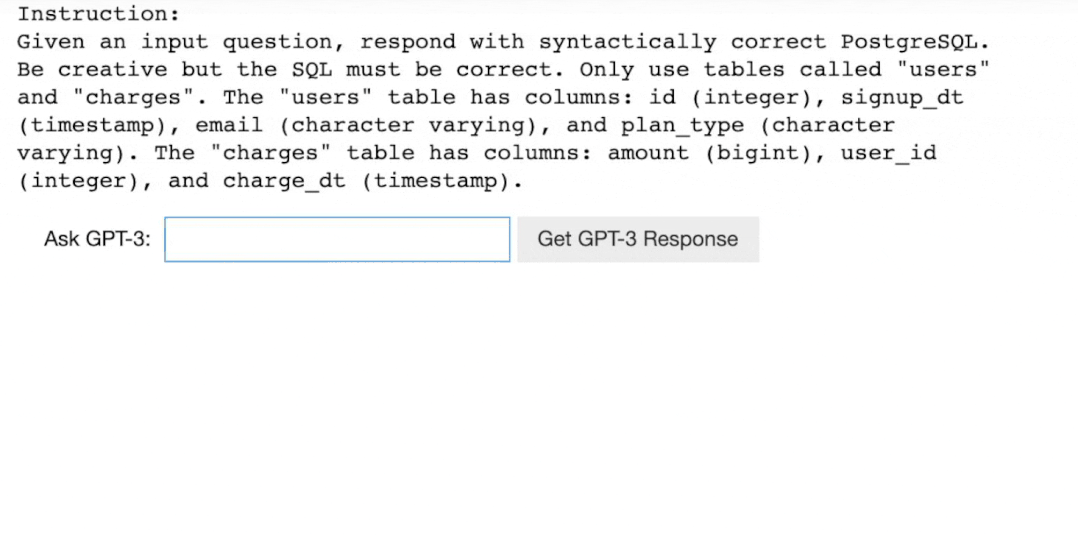Temperature为.2时 GPT-3：

``SELECT SUM(CASE WHEN signup_dt >= now() - interval '6 months' THEN amount ELSE 0 END) AS revenue FROM charges ``### 结论

GPT-3 实例会根据简单的英语问题，将其转换为数据库真正有效的SQL。它不总是完美，对“增长率”或“百分比”等复杂的概念仍然需要一些人工帮助，但是它对我的日常工作是有用，对于简单的SQL，GPT-3 能帮忙减轻我的负担，并且对于不熟悉 SQL 语法的初学者很有用。11 0